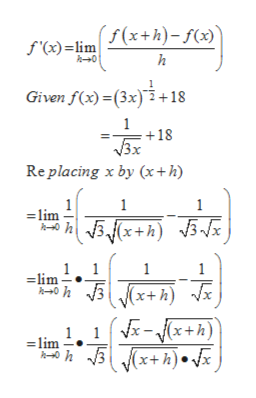Compute f'(x) using the limit definition if f(x) = (3x)-1/2 + 18(Use symbolic notation and fractions where needed.f'(x)

Questionhelp_outlineImage TranscriptioncloseCompute f'(x) using the limit definition if f(x) = (3x)-1/2 + 18 (Use symbolic notation and fractions where needed. f'(x) fullscreen
Step 1

Find f’(x) using limit definition for function

Step 2

By definit...help_outlineImage Transcriptionclosef'(x) =lim(x+h)-f(x) +0 h Given f(x)(3x)18 1 +18 Replacing x by (x+ h) 1 1 =lim 1 lim 1 (x+h) 1 lim V (x+h).x fullscreen

Want to see the full answer?

See Solution

Want to see this answer and more?

Our solutions are written by experts, many with advanced degrees, and available 24/7

See Solution
Tagged in

Limits